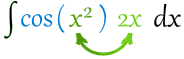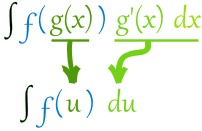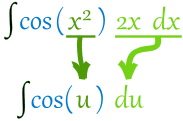# Integration by Substitution

“Integration by Substitution” (also called “u-Substitution” or “The Reverse Chain Rule”) is a method to find an integral, but only when it can be set up in a special way.

The first and most vital step is to be able to write our integral in this form:Note that we have $g(x)$ and its derivative $g'(x)$

Like in this example:Here $f=cos$, and we have $g=x^2$  and its derivative $2x$.

This integral is good to go!

When our integral is set up like that, we can do this substitution:Then we can integrate $f(u)$, and finish by putting $g(x)$ back as $u$.

## Substitution Rule## Example Integration by Substitution

Example 1. Find the integral $$\int \cos(x^2) 2x dx$$

We know (from above) that it is in the right form to do the substitution: